• +91 9971497814
• info@interviewmaterial.com

# Chapter 14- Oscillations Interview Questions Answers

### Related Subjects

Question 1 :
Which of the following examples represent periodic motion?
(a) A swimmer completing one (return) trip from one bank of a river to the other and back.
(b) A freely suspended bar magnet displaced from its N-S direction and released.
(c) A hydrogen molecule rotating about its centre of mass.
(d) An arrow released from a bow.

(a) It is not a periodic motion. Though the motion of a swimmer is to and fro but will not have a definite period.
(b) Since a freely suspended magnet if once displaced from N-S direction and released, it oscillates about this position, it is a periodic motion.
(c) The rotating motion of a hydrogen molecule about its centre of mass is periodic.
(d) Motion of an arrow released from a bow is non-periodic

Question 2 :
Which of the following examples represent (nearly) simple harmonic motion and which represent
periodic but not simple harmonic motion?
(a) the rotations of earth about its axis.
(b) motion of an oscillating mercury column in a U-tube.
(c) motion of a ball bearing inside a smooth curved bowl, when released from a point slightly above the lowermost point.
(d) general vibrations of a polyatomic molecule about its equilibrium position.

(a) Since the rotation of earth is not to and fro motion about a fixed point, thus it is periodic but not S.H.M.
(b) It is S.H.M.
(c) It is S.H.M.
(d) General vibrations of a polyatomic molecule about its equilibrium position is periodic but non SHM. In fact, it is a result of superposition of SHMs executed by individual vibrations of atoms of the molecule.

Question 3 : Fig. depicts four x-t plots for linear motion of a particle. Which of ike plots represent periodic motion? What is the period of motion (in case of periodic motion)?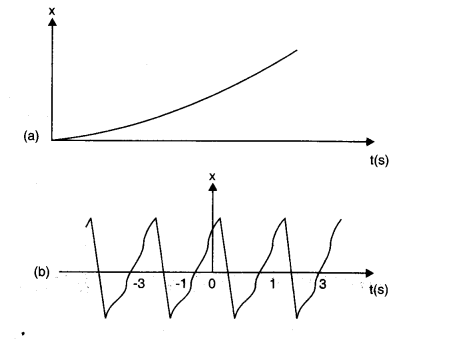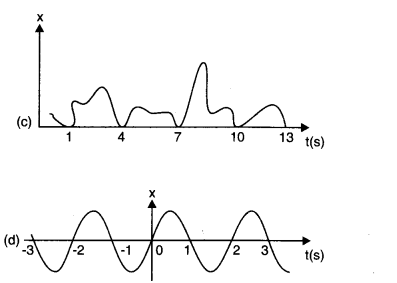Answer 3 : Figure (b) and (d) represent periodic motions and the time period of each of these is 2 seconds, (a) and (c) are non-periodic motions.

Question 4 : Which of the following functionof time represent (a) simple harmonic, (b) periodic but not simple harmonic,and (c) non-periodic motion? Give period for each case of periodic motion (tois any positive constant).
(a) sin wt – cos wt (b) sin2 wt(c) 3 cos -2 cos (π/4-2 wt) (d) cos wt + cos 3 wt + cos 5 wt
(e) exp (- w2t2) (f) 1 + wt + w2t2.

Answer 4 : Thefunction will represent a periodic motion, if it is identically repeated aftera fixed interval of time and will represent S.H.M if it can be written uniquelyin the form of a cos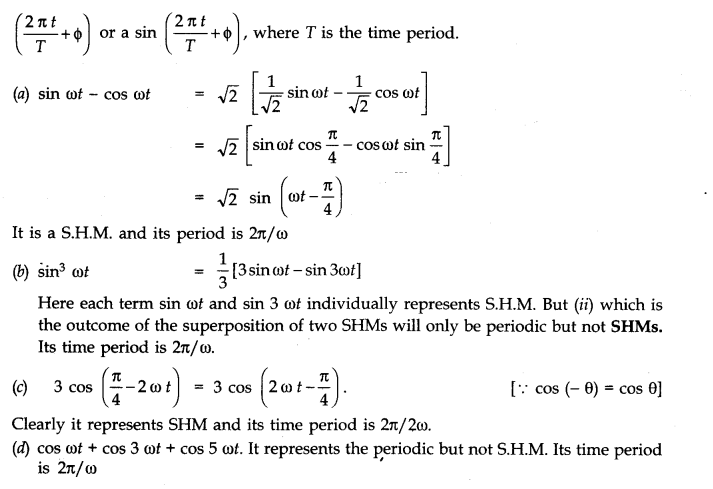(e) e-w2t2 . It is an exponential function which never repeats itself. Therefore it represents non-periodic motion.
(f) 1 + wt + w2t2 also represents non periodic motion.

Question 5 :
A particle is in linear simple harmonic motion between two points, A and B, 10 cm apart. Take the direction from A to B as the positive direction and give the signs of velocity, acceleration and force on the particle when it is
(a) at the end A,
(b) at the end B,
(c) at the mid-point of AB going towards A,
(d) at 2 cm away from B going towards A,
(e) at 3 cm away from A going towards B, and (f)  at 4 cm away from B going towards A.

Answer 5 : In the fig. (given below), the points A and B, 10 cm apart, are the extreme positions of the particle in SHM, and the point O is the mean position. The direction from A to B is positive, as indicated.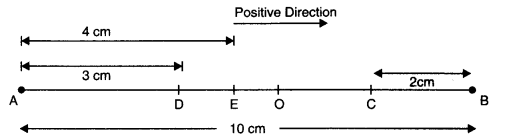(a) At the end A, i.e., extreme position, velocity is zero, acceleration and force are directed towards O and are positive.
(b) At the end B, i.e., second extreme position, velocity is zero whereas the acceleration and force are directed towards the point O and are negative.
(c) At the mid point O, while going towards A, velocity is negative and maximum. The acceleration and force both are zero.
(d) At 2 cm away from B, that is, at C and going towards A: v is negative; acceleration and F, being directed towards O, are also negative.
(e) At 3 cm away from A, that is, at D and going towards B: v is positive; acceleration and F, being directed towards O, are also positive.
(f)  At a distance of 4 cm away from A and going towards A, velocity is directed along BA, therefore, it is positive. Since acceleration and force are directed towards OB, both of them are positive.

Question 6 :
Which of the following relationships between the acceleration a and the displacement x of a particle involve simple harmonic motion?
(a) a = 0.7x (b) a = – 200 x2
(c) a = – 10x (d) a = 100 x3

Answer 6 : Only (c) i.e., a = – 10x represents SHM. This is because acceleration is proportional and opposite to displacement (x).

Question 7 : . The motion of a particle executing simple harmonic motion is described by the displacement function. x(t) = A cos (wt +Ф ).If the initial (t = 0) position of the particle is 1 cm and its initial velocity is w cm/s, what are its amplitude and initial phase angle? The angular frequency of the particle is π s-1. If instead of the cosine function, we choose the sine function to describe the SHM: x = B sin (wt + α), what are the amplitude and initial phase of the particle with the above initial conditions?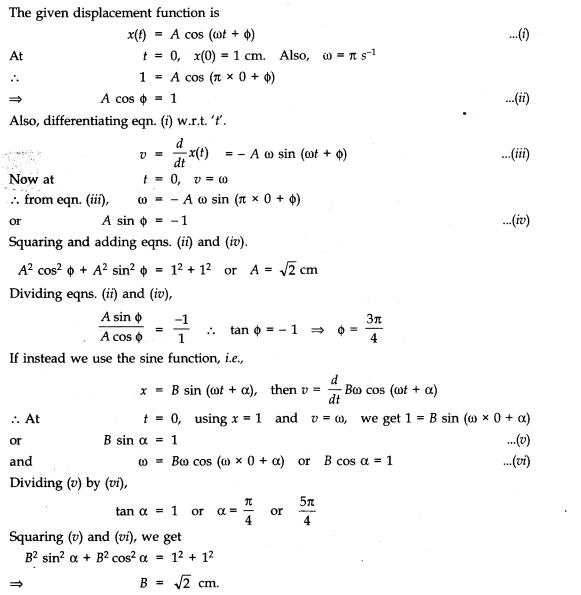Question 8 : A spring balance has a scale that reads from 0 to 50 kg. The length of the scale is 20 cm. A body suspended from this balance, when displaced and released, oscillates with a period of 0.6 s. What is the weight of the body?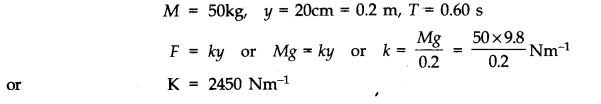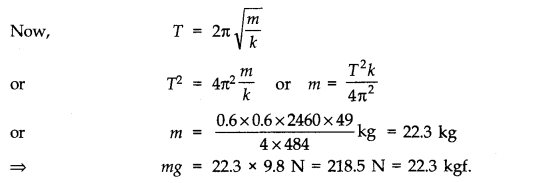Question 9 : A spring having with a spring constant 1200 Nm-1 is mounted on a horizontal table as shown in Fig. A mass of 3 kg is attached to the free end of the spring. The mass is then pulled sideways to a distance of 2.0 cm and released. Determine (i) the frequency of oscillations, (ii) maximum acceleration of the mass, and (iii) the maximum speed of the mass.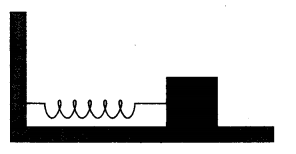Answer 9 :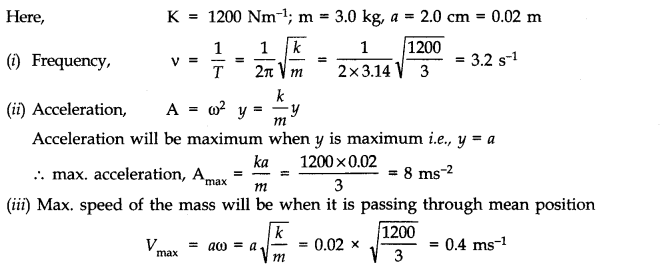Question 10 : In Exercise 9, let us take the position of mass when the spring is unstreched as x = 0, and the direction from left to right as the positive direction of x – axis. Give x as a function of time t for the oscillating mass if at the moment we start the stopwatch (t = 0), the mass is (a)at the mean position,(b)at the maximum stretched position, and (c)at the maximum compressed position.In what way do these functions for SHM differ from each other, in frequency, in amplitude or the initial phase?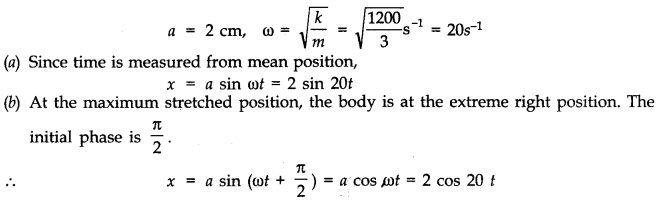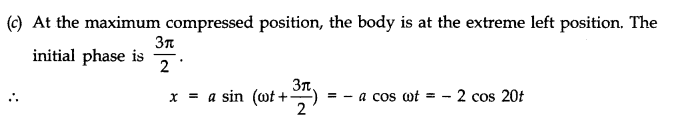Note: The functions neither differ in amplitude nor in frequency . They differ only in initial phase.

Todays Deals### Chapter 14- Oscillations Contributorskrishan

Name:
Email:

# Latest News# 9000 interview questions in different categories